Figure of Merit of a galvanometer

# Objective

To determine the resistance of a galvanometer by half deflection method and to find its figure of merit.

# Theory

## Galvanometer

A galvanometer is a device used to detect feeble electric currents in a circuit. It consists of a coil suspended between the poles of a powerful magnet. As current passes through the coil, it deflects. It can be detected from the deflection on galvanometer needle. The deflection is proportional to the current passed through it.

## Resistance of galvanometer by half deflection method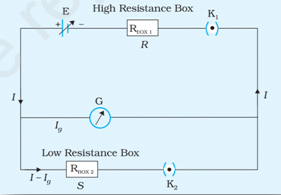Here, current will flows through th circuit when key k1 is closed and k2 is open. The current flowing through the galvanometer is proportional to the deflection in it.Where, E - emf f the cell

R - resistance from the resistance box
G - galvanometer resistance for current I
θ - galvanometer deflection for current I
k – proportionality constant.

When k2 is closed and by adjusting the shunt resistance S, we can make galvanometer deflection as θ/2.

Then the current in the circuit is ;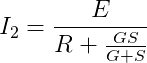Now, a fraction, S/ (G+S) of the current in the circuit is flows through the galvanometer, which is given by,Now, from the above relations, we can get the resistance of the given galvanometer as,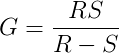## Figure of merit of a galvanometer

Figure of merit is in general is the numerical value representing the degree of effectiveness or efficiency of an instrument approximated by different estimation techniques.

The figure of merit of a galvanometer is the current required to produce a deflection of one division in the galvanometer scale. It is represented by the letter k, and is given as,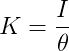or  ,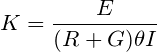# Learning Outcomes

• Students understand the various components used in the experiment.
• Students learn the concept, ‘figure of merit’.
• Students are able to construct circuits based on circuit diagrams.

Cite this Simulator: